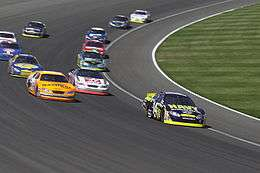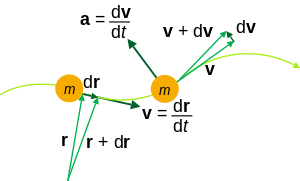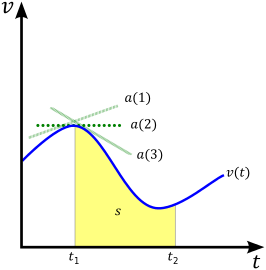# Velocity

VelocityAs a change of direction occurs while the racing cars turn on the curved track, their velocity is not constant.
Common symbols
v, v
SI unit m/s

The velocity of an object is the rate of change of its position with respect to a frame of reference, and is a function of time. Velocity is equivalent to a specification of its speed and direction of motion (e.g. 60 km/h to the north). Velocity is an important concept in kinematics, the branch of classical mechanics that describes the motion of bodies.

Velocity is a physical vector quantity; both magnitude and direction are needed to define it. The scalar absolute value (magnitude) of velocity is called "speed", being a coherent derived unit whose quantity is measured in the SI (metric) system as metres per second (m/s) or as the SI base unit of (m⋅s−1). For example, "5 metres per second" is a scalar, whereas "5 metres per second east" is a vector.

If there is a change in speed, direction or both, then the object has a changing velocity and is said to be undergoing an acceleration.

## Constant velocity vs accelerationKinematic quantities of a classical particle: mass m, position r, velocity v, acceleration a.

To have a constant velocity, an object must have a constant speed in a constant direction. Constant direction constrains the object to motion in a straight path thus, a constant velocity means motion in a straight line at a constant speed.

For example, a car moving at a constant 20 kilometres per hour in a circular path has a constant speed, but does not have a constant velocity because its direction changes. Hence, the car is considered to be undergoing an acceleration.

## Distinction between speed and velocity

Speed describes only how fast an object is moving, whereas velocity gives both how fast and in what direction the object is moving. If a car is said to travel at 60 km/h, its speed has been specified. However, if the car is said to move at 60 km/h to the north, its velocity has now been specified.

The big difference can be noticed when we consider movement around a circle. When something moves in a circle and returns to its starting point, its average velocity is zero but its average speed is found by dividing the circumference of the circle by the time taken to move around the circle. This is because the average velocity is calculated by only considering the displacement between the starting and the end points while the average speed considers only the total distance traveled.

## Equation of motion

Main article: Equation of motion

### Average velocity

Velocity is defined as the rate of change of position with respect to time, which may also be referred to as the instantaneous velocity to emphasize the distinction from the average velocity. In some applications the "average velocity" of an object might be needed, that is to say, the constant velocity that would provide the same resultant displacement as a variable velocity in the same time interval, v(t), over some time period Δt. Average velocity can be calculated as:The average velocity is always less than or equal to the average speed of an object. This can be seen by realizing that while distance is always strictly increasing, displacement can increase or decrease in magnitude as well as change direction.

In terms of a displacement-time (x vs. t) graph, the instantaneous velocity (or, simply, velocity) can be thought of as the slope of the tangent line to the curve at any point, and the average velocity as the slope of the secant line between two points with t coordinates equal to the boundaries of the time period for the average velocity.

The average velocity is the same as the velocity averaged over time – that is to say, its time-weighted average, which may be calculated as the time integral of the velocity:where we may identifyand### Instantaneous velocityExample of a velocity vs. time graph, and the relationship between velocity v on the y-axis, acceleration a (the three green tangent lines represent the values for acceleration at different points along the curve) and displacement s (the yellow area under the curve.)

If we consider v as velocity and x as the displacement (change in position) vector, then we can express the (instantaneous) velocity of a particle or object, at any particular time t, as the derivative of the position with respect to time:From this derivative equation, in the one-dimensional case it can be seen that the area under a velocity vs. time (v vs. t graph) is the displacement, x. In calculus terms, the integral of the velocity function v(t) is the displacement function x(t). In the figure, this corresponds to the yellow area under the curve labeled s (s being an alternative notation for displacement).Since the derivative of the position with respect to time gives the change in position (in metres) divided by the change in time (in seconds), velocity is measured in metres per second (m/s). Although the concept of an instantaneous velocity might at first seem counter-intuitive, it may be thought of as the velocity that the object would continue to travel at if it stopped accelerating at that moment.

### Relationship to acceleration

Although velocity is defined as the rate of change of position, it is often common to start with an expression for an object's acceleration. As seen by the three green tangent lines in the figure, an object's instantaneous acceleration at a point in time is the slope of the line tangent to the curve of a (v vs. t graph at that point. In other words, acceleration is defined as the derivative of velocity with respect to time:From there, we can obtain an expression for velocity as the area under an acceleration vs. time (a vs. t) graph. As above, this is done using the concept of the integral:#### Constant acceleration

In the special case of constant acceleration, velocity can be studied using the suvat equations. By considering a as being equal to some arbitrary constant vector, it is trivial to show thatwith v as the velocity at time t and u as the velocity at time t = 0. By combining this equation with the suvat equation x = ut + at2/2, it is possible to relate the displacement and the average velocity by.

It is also possible to derive an expression for the velocity independent of time, known as the Torricelli equation, as follows:where v = |v| etc.

The above equations are valid for both Newtonian mechanics and special relativity. Where Newtonian mechanics and special relativity differ is in how different observers would describe the same situation. In particular, in Newtonian mechanics, all observers agree on the value of t and the transformation rules for position create a situation in which all non-accelerating observers would describe the acceleration of an object with the same values. Neither is true for special relativity. In other words, only relative velocity can be calculated.

### Quantities that are dependent on velocity

The kinetic energy of a moving object is dependent on its velocity and is given by the equationignoring special relativity, where Ek is the kinetic energy and m is the mass. Kinetic energy is a scalar quantity as it depends on the square of the velocity, however a related quantity, momentum, is a vector and defined byIn special relativity, the dimensionless Lorentz Factor appears frequently, and is given bywhere γ is the Lorentz factor and c is the speed of light.

Escape velocity is the minimum speed a ballistic object needs to escape from a massive body such as Earth. It represents the kinetic energy that, when added to the object's gravitational potential energy, (which is always negative) is equal to zero. The general formula for the escape velocity of an object at a distance r from the center of a planet with mass M iswhere G is the Gravitational constant and g is the Gravitational acceleration. The escape velocity from Earth's surface is about 11 200 m/s, and is irrespective of the direction of the object.

## Relative velocity

Main article: Relative velocity

Relative velocity is a measurement of velocity between two objects as determined in a single coordinate system. Relative velocity is fundamental in both classical and modern physics, since many systems in physics deal with the relative motion of two or more particles. In Newtonian mechanics, the relative velocity is independent of the chosen inertial reference frame. This is not the case anymore with special relativity in which velocities depend on the choice of reference frame.

If an object A is moving with velocity vector v and an object B with velocity vector w, then the velocity of object A relative to object B is defined as the difference of the two velocity vectors:Similarly the relative velocity of object B moving with velocity w, relative to object A moving with velocity v is:Usually the inertial frame is chosen in which the latter of the two mentioned objects is in rest.

### Scalar velocities

In the one-dimensional case, the velocities are scalars and the equation is either:, if the two objects are moving in opposite directions, or:, if the two objects are moving in the same direction.

## Polar coordinates

In polar coordinates, a two-dimensional velocity is described by a radial velocity, defined as the component of velocity away from or toward the origin (also known as velocity made good), and an angular velocity, which is the rate of rotation about the origin (with positive quantities representing counter-clockwise rotation and negative quantities representing clockwise rotation, in a right-handed coordinate system).

The radial and angular velocities can be derived from the Cartesian velocity and displacement vectors by decomposing the velocity vector into radial and transverse components. The transverse velocity is the component of velocity along a circle centered at the origin.whereis the transverse velocityis the radial velocity.

The magnitude of the radial velocity is the dot product of the velocity vector and the unit vector in the direction of the displacement.whereis displacement.

The magnitude of the transverse velocity is that of the cross product of the unit vector in the direction of the displacement and the velocity vector. It is also the product of the angular speedand the magnitude of the displacement.such thatAngular momentum in scalar form is the mass times the distance to the origin times the transverse velocity, or equivalently, the mass times the distance squared times the angular speed. The sign convention for angular momentum is the same as that for angular velocity.whereis massThe expressionis known as moment of inertia. If forces are in the radial direction only with an inverse square dependence, as in the case of a gravitational orbit, angular momentum is constant, and transverse speed is inversely proportional to the distance, angular speed is inversely proportional to the distance squared, and the rate at which area is swept out is constant. These relations are known as Kepler's laws of planetary motion.

## Notes

1. Wilson, Edwin Bidwell (1901). Vector analysis: a text-book for the use of students of mathematics and physics, founded upon the lectures of J. Willard Gibbs. p. 125. This is the likely origin of the speed/velocity terminology in vector physics.
2. Basic principle

## References

• Robert Resnick and Jearl Walker, Fundamentals of Physics, Wiley; 7 Sub edition (June 16, 2004). ISBN 0-471-23231-9.Wikimedia Commons has media related to Velocity.